# IB DP Physics: SL复习笔记2.2.1 Free-Body Diagrams

### Vectors

• In physics, during force interactions, it is common to represent situations as simply as possible without losing information
• When considering force interactions objects can be represented as point particles
• These point particles should be placed at the center of mass of the object
• Force vectors that act upon that objects should be drawn with their tail on that point particle
• The length of the force vector corresponds to its strength
• The longer the vector, the greater the force magnitude
• The below example shows the forces acting on an object when pushed to the right over a rough surface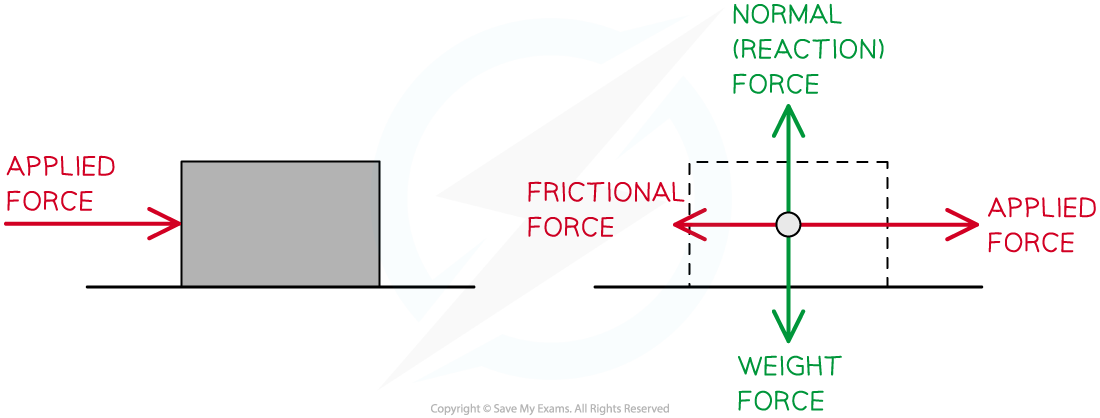Point particle representation of the forces acting on a moving object

• The below example shows an object sitting on a slope in equilibrium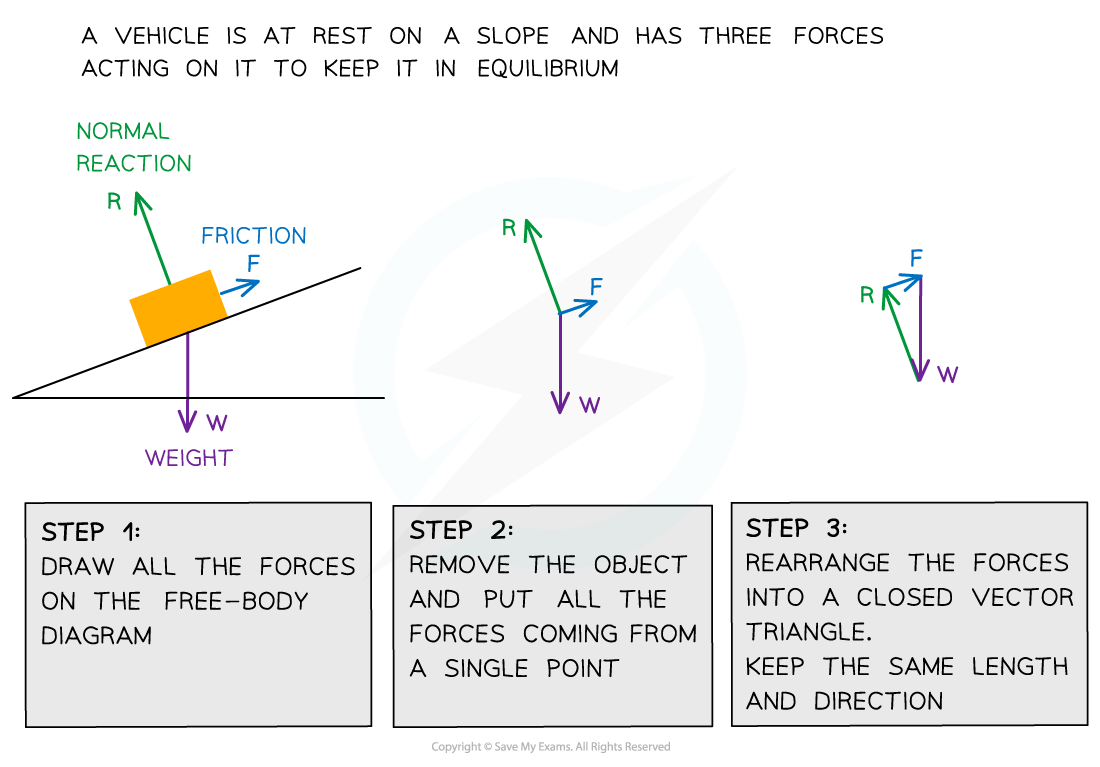Three forces on an object in equilibrium form a closed vector triangle

• The below example shows the forces acting on an object suspended from a stationary rope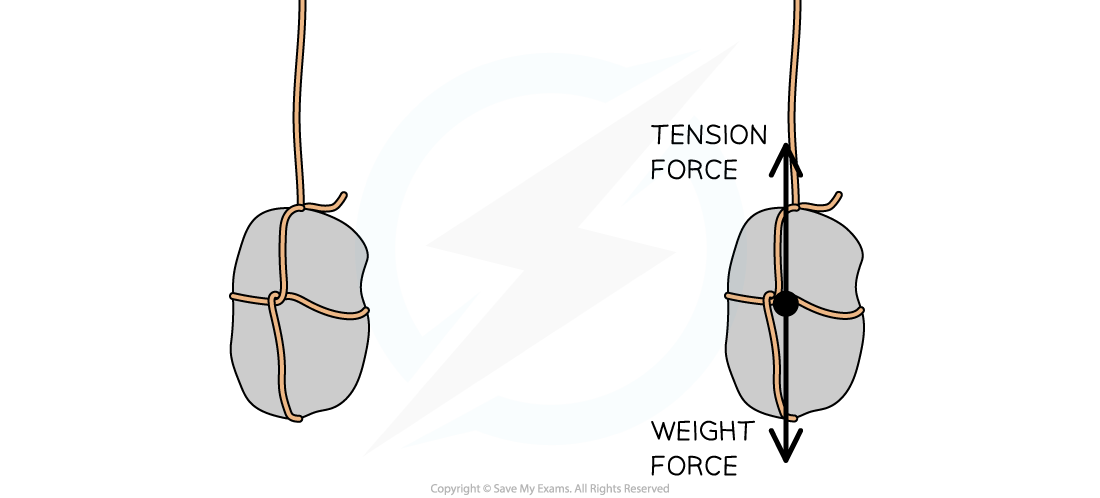Free-body diagram of an object suspended from a stationary rope

#### Exam Tip

When labeling force vectors, it is important to use conventional and appropriate naming or symbols such as:

• w or Weight force or mg
• N or R for normal reaction force (depending on your local context either of these could be acceptable)

Using unexpected notation can lead to losing marks so try to be consistent with expected conventions.

### Free-Body Diagrams

• Free body diagrams are useful for modeling the forces that are acting on an object
• Each force is represented as a vector arrow, where each arrow:
• Is scaled to the magnitude of the force it represents
• Points in the direction that the force acts
• Is labelled with the name of the force it represents or an appropriate symbol
• Free body diagrams can be used:
• To identify which forces act in which plane
• To resolve the net force in a particular direction
• The rules for drawing a free-body diagram are the following:
• Rule 1: Draw a point in the centre of mass of the body
• Rule 2: Draw the body free from contact with any other object
• Rule 3: Draw the forces acting on that body using vectors with correct direction and proportional lengthFree body diagrams can be used to show the various forces acting on objects

• You must be able to apply the following forces with their symbols to free-body diagrams:
• Weight (W)
• Tension (T)
• Normal Reaction Force (N)
• Upthrust (U)
• Frictional Forces (Fr)

#### Worked Example

Draw free-body diagrams for the following scenarios:

a) A picture frame hanging from a nail

b) A box sliding down a slope

Part (a)

A picture frame hanging from a nail:• The size of the arrows should be such that the 3 forces would make a closed triangle as they are balanced

Part (b)

A box sliding down a slope: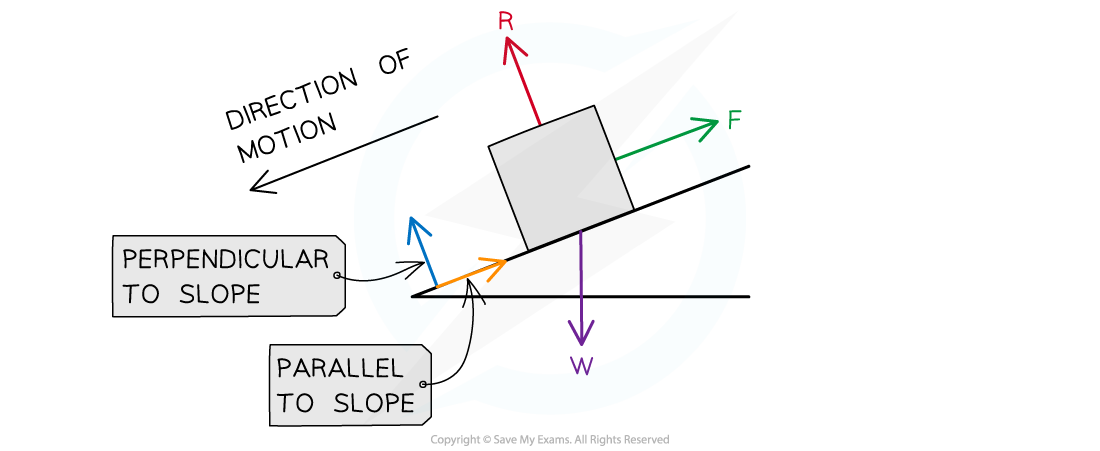• There are three forces acting on the box:
• The normal contact force, R, acts perpendicular to the slope
• Friction, F, acts parallel to the slope and in the opposite direction to the direction of motion
• Weight, W, acts down towards the Earth

#### Worked Example

Draw a free-body diagram of a toy sailboat with weight 30 N floating in water that is being pulled to the right by an applied force of 35 N with a total resistive force of 5 N.

Step 1: Draw the object in a simplified diagram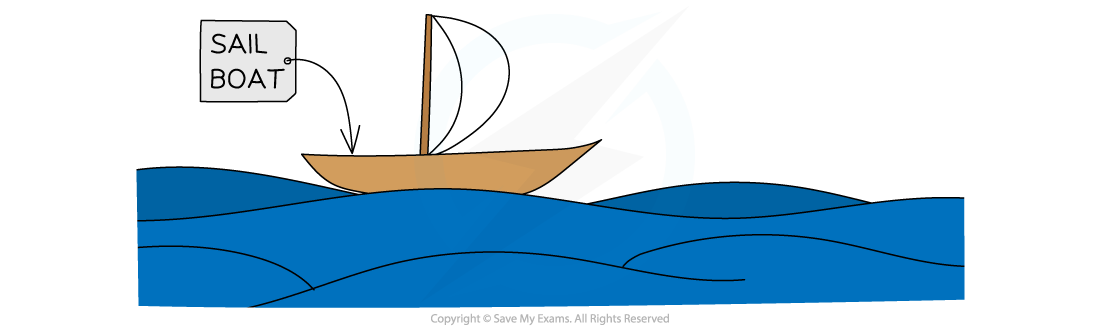Step 2: Identify all of the forces acting upon the object in the question, including any forces that may be implied

• Weight: 30 N down
• Upthrust from the water (since the object is floating): 30 N up
• Applied force: 35 N to the right
• Resistive force: 5 N to the left

Step 3: Draw in all of the force vectors (arrows), making sure the arrows start at the object and are directed away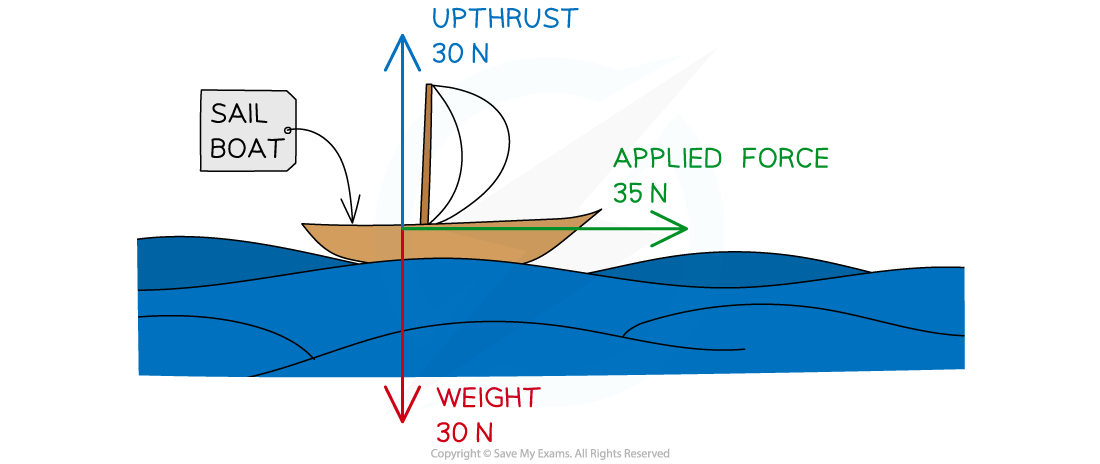• An approximation can be made as to the final resultant force due to all of the forces
• Decide whether the resultant force is approximately up or down
• Decide whether the resultant force is approximately left or right
• For example, the resultant force is directed up and to the right